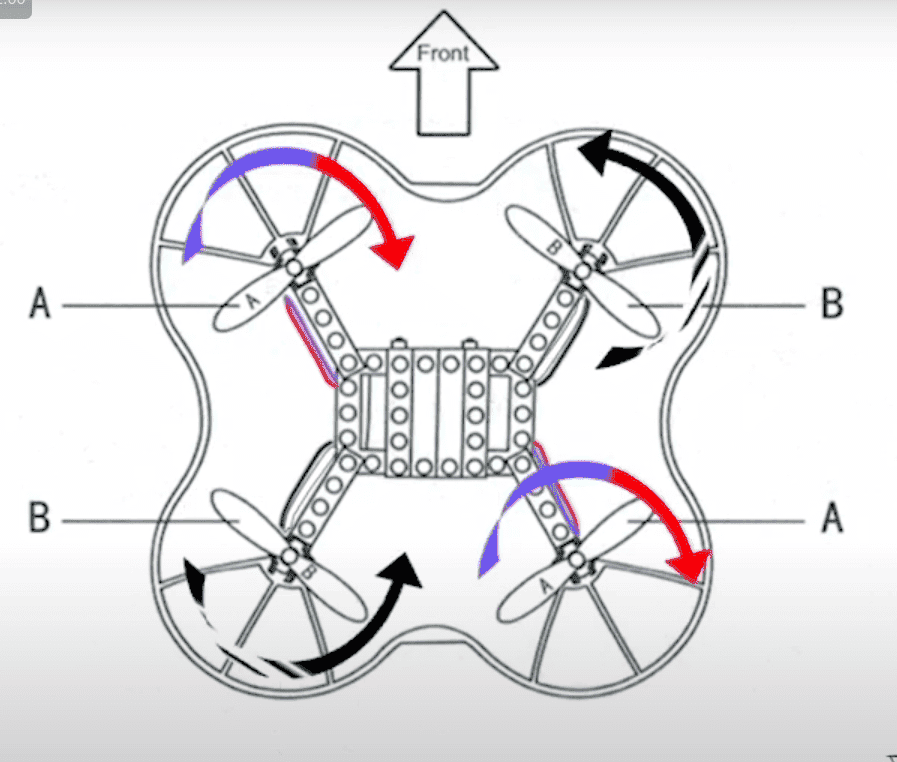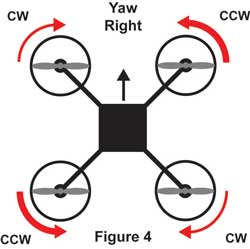# Conservation of angular momentum and its counterpart for linear momentum

Hi, I have just joined the forum. Thank you all for being a part of such places so that people like me can get answers to the questions on their minds!

---------------------------

I have been trying to understand how a quadcopter yaws. Referring to the figure below which is bird's eye view of a quadcopter:While the quadcopter is just hovering, the net angular momentum of the system is zero, since the angular momentums of motor set A and motor set B will be equal in magnitude and opposite in direction. So, far, so good.

Now, if I increase the rpm, so the angular velocity(ω) of the propellers, of the motor set B; then they will produce more(in magnitude) angular momentum w.r.t motor set A. So, the total angular momentum of the system will not remain at 0 but will increase towards upward(out of the page) direction. At this point, the phenomenon which causes quad to yaw comes into play, the quadcopter starts to rotate in the opposite direction of motor set B, i.e clockwise, to preserve previous net angular momentum of the system, which was 0. So, at this point, we call it conservation of angular momentum of the system.

Am I consistent with the laws of physics so far?

If I am, then, I get confused when I think the same conservation thing for linear momentum. So, we have our car at rest. So the initial linear momentum of the system is 0 since the speed is 0 (v=0). Then we increase gas pedal, and car starts to move. So the final linear momentum of the car is not 0 anymore. But, why, something similar to the quad case does not happen? In that case, system was counteracting the change in the momentum. But our car just gained momentum.

I know this part sounds very silly, but, I think I have some misunderstandings somewhere. So, any help would be appreciated.

Last edited:

jbriggs444
Homework Helper
I think the same conservation thing for linear momentum. So, we have our car at rest. So the initial linear momentum of the system is 0 since the speed is 0 (v=0). Then we increase gas pedal, and car starts to move. So the final linear momentum of the car is not 0 anymore. But, why, something similar to the quad case does not happen? In that case, system was counteracting the change in the momentum. But our car just gained momentum.
You do have a similar thing.

The car pushes on the ground. The car moves forward. The ground moves backward. The ground is very big and awfully heavy. So it does not move very fast.

•etotheipi and Lnewqban
Lnewqban
Gold Member
The drone does not have much resistance to yaw.
The car is trapped between gravity and the road.
The wheels try to lift the car (pitch up), but it is too heavy, therefore, they move it forward instead.

Motocross riders control the pitching attitude of the bike while in the air and for landing by applying brakes or accelerator, achieving a pitching reaction that way.
That is similar to what happens to the drone, but in a vertical plane.

Last edited:
A.T.
No, if I increase the rpm, so the angular velocity(ω) of the propellers, of the motor set B; then they will produce more(in magnitude) angular momentum w.r.t motor set A. So, the total angular momentum of the system will not remain at 0 but will increase towards upward(out of the page) direction. At this point, the phenomenon which causes quad to yaw comes into play, the quadcopter starts to rotate in the opposite direction of motor set B, i.e clockwise, to preserve previous net angular momentum of the system, which was 0. So, at this point, we call it conservation of angular momentum of the system.
This is not a satellite in vacuum. The rotors experience an aerodynamic torque from the drag of the blades. If the CCW rotors spin faster than the CW rotors, there is a CW net external torque on from the air on the whole quad-copter.

So, we have our car at rest. So the initial linear momentum of the system is 0 since the speed is 0 (v=0). Then we increase gas pedal, and car starts to move. So the final linear momentum of the car is not 0 anymore. But, why, something similar to the quad case does not happen? In that case, system was counteracting the change in the momentum. But our car just gained momentum.
There is an external force on the car from the ground.

The wheels try to lift the car (pitch up), but it is too heavy, therefore, they move it forward instead.

For a car going in forward direction, the wheels are generating an angular momentum(or torque) pointed towards the left. So, for balancing, the body of the car wants to generate a torque towards right, which could be obtained if the car were rotating so that it pitches its nose up.

That's why you said pitch up, right?

You do have a similar thing.

The car pushes on the ground. The car moves forward. The ground moves backward. The ground is very big and awfully heavy. So it does not move very fast.

Interesting... So, I should consider car + earth surface as my system, then a component in the system (car) may not be preserved its linear momentum, but for the whole system, it is preserved since earth surface is moving twords the opposite way with lots of mass but very little speed. Something like this?

Last edited:
•jbriggs444
jbriggs444
Homework Helper
but for the whole system, it is preserved since earth surface is moving other way with lots of mass but very little speed. Something like this?
Yes, that's it.

•muyustan
Yes, that's it.

Then the thing which aparts my quadcopter example from the car example is the fact that quad motors(by propellers) are better in being able to influence their systems (quadcopter + air) compared to car motors(by wheels) and their systems (car + earth). And that is probably because car motors have to overcome whole mass of the earth, while quadcopter motors have to overcome air.

It kind of makes sense now.

A.T.
...a component in the system (car) may not be preserved its linear momentum, but for the whole system, ...
Linear/angular momentum are conserved for isolated systems. Neither the quadcopter nor the car are isolated.

What you described regarding the yaw fits better to satellites, which can re-orient themselves using reaction wheels.

Linear/angular momentum are conserved for isolated systems. Neither the quadcopter nor the car are isolated.

But, what the guys rotating themselves with a rotating bicycle wheel are demonstrating then? Isn't it conservation of angular momentum? Are they isolated?

A.T.
But, what the guys rotating themselves with a rotating bicycle wheel are demonstrating then? Isn't it conservation of angular momentum? Are they isolated?

The are partially isolated. The turntable eliminates the external torques around one axis, so you can assume momentum conservation around that axis.

But you cannot assume that for the quadcopter with CCW rotors spinning at different RPM than the CW rotors, because the drag-torques on the rotors are RPM dependent. This implies a net external torque by the air around the vertical axis.

The are partially isolated. The turntable eliminates the external torques around one axis, so you can assume momentum conservation around that axis.

But you cannot assume that for the quadcopter with CCW rotors spinning at different RPM than the CW rotors, because the drag-torques on the rotors are RPM dependent. This implies a net external torque by the air around the vertical axis.

Hmmm, so how correct is summarizing the situation as follows?

CW motors experience a drag force from the air which will result in a torque which will result in a rotation CCW around the vertical axis(origin at center of gravity of the quadcopter). So as rpm of CW motors increase relative to CCW motors, the drag force increases, thus torque in CCW increases and rotates the drone CCW.

Am I even close to the point?

If so, other than relating this to angular momentum conservation, I at least can say that I understood the yaw mechanics.

A.T.
CW motors experience a drag force from the air which will result in a torque which will result in a rotation CCW around the vertical axis(origin at center of gravity of the quadcopter).
The horizontal force on each rotor is zero, and doesn't create any vertical torque around the the center of gravity of the quadcopter. But the blades of the rotors experience a drag force, which results in a torque around each rotor center. The total vertical torque on the quadcopter is the sum of the vertical torques around each rotor center plus the (zero) torque from the horizontal force at each rotor center.

So as rpm of CW motors increase relative to CCW motors, the drag force increases, thus torque in CCW increases and rotates the drone CCW.
Yes, but again: It's the drag-forces on each blade, or drag-torques on each rotor. There is no drag-force per rotor.

If so, other than relating this to angular momentum conservation, I at least can say that I understood the yaw mechanics.
The inertial mechanism you described can contribute to yaw, but is not necessary. The quadcopter could yaw using this RPM-difference-method, even if the spinning parts were masses. I don't know what fraction each effect contributes to yaw for a typical quadcopter.

Last edited:
•muyustan
Lnewqban
Gold Member
For a car going in forward direction, the wheels are generating an angular momentum(or torque) pointed towards the left. So, for balancing, the body of the car wants to generate a torque towards right, which could be obtained if the car were rotating so that it pitches its nose up.

That's why you said pitch up, right?
Yes, although the location of the center of mass and its acceleration contributes more to that nose up pitch that you see each time a car accelerates.
Perhaps, the reactive torque of a powerful V8 engine is a better example.

Also, compensating for the changes of rpm and the horizontal component of drag (associate to lift and friction) that would induce an undesired yaw movement, is one of the reasons for the existence of the tail rotor of helicopters.

Copied from
http://www.thedronesmag.com/the-physics-of-multirotor-drone-flight/

"The next control movement often confuses those who are new to multirotors. How exactly does a quad yaw or rotate left and right? In a quad, as seen in the previous figures, two of the motors are spinning clockwise while the opposite two motors are spinning counter-clockwise. In this condition the natural torque tendencies of one pair of motors is cancelled out by the torque of the opposite pair and the quad does not rotate. Looking at Figure 4, to make the quad rotate to the right thetwo counter-clockwise
rotating motors are sped up, while the two clockwise rotating motors are slowed down by the same amount. This keeps the total lift generated the same, because the amount of thrust gained by the faster turning motors is the same as the amount of thrust lost by the slower turning motors. However, the torque moment generated by the counterclockwise motor increases while the torque moment of the clockwise motors decreases. This results is a net torque increase that causes the entire quad frame to rotate clockwise. Conversely, to yaw to the left , the two clockwise rotating motors are sped up while the two counter-clockwise motors are slowed down and the net torque difference rotates the quad frame to the left."